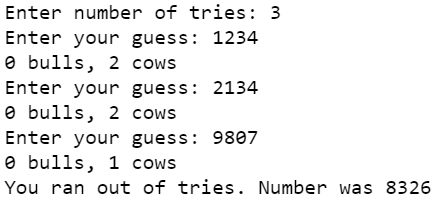# Python – Cows and Bulls game

Cows and Bulls is a pen and paper code-breaking game usually played between 2 players. In this, a player tries to guess a secret code number chosen by the second player. The rules are as follows:

• A player will create a secret code, usually a 4-digit number.  This number should have no repeated digits.
• Another player makes a guess (4 digit number) to crack the secret number. Upon making a guess, 2 hints will be provided- Cows and Bulls.
• Bulls indicate the number of correct digits in the correct position and cows indicates the number of correct digits in the wrong position. For example, if the secret code is 1234 and the guessed number is 1246 then we have 2 BULLS (for the exact matches of digits 1 and 2) and 1 COW (for the match of digit 4 in the wrong position)
• The player keeps on guessing until the secret code is cracked. The player who guesses in the minimum number of tries wins.

Explanation:

Let’s see a sample run for better understanding.

```Secret Code: 3768

Guess: 1234
Response: 0 bulls, 1 cow
Guess: 5678
Response: 1 bull, 2 cows
Guess: 1678
Response: 1 bull, 2 cows
Guess: 3678
Response: 2 bulls, 2 cows
Guess: 3148
Response: 2 bulls, 0 cows
Guess: 3768
You guessed right!```

Approach:

To create this game in Python, the computer generates a secret code and the user will have to guess the code. Break it down into these blocks:

• Generate a secret code- Generate a random 4-digit number and check that it does not have any repeated digits.
• Generate hint or response- Take the generated 4-digit secret number and the guessed number (input). Find the common digits with exact matches (bulls) and the common digits in the wrong position (cows). Repeat with each guess until you have 4 bulls (an exact match) or you run out of tries.

Constraint: The secret code and the guessed code should be of 4-digits (between 1000 and 9999) and have no repeated numbers.

Program:

## Python

 `# Import required module ` `import` `random  ` ` `  `# Returns list of digits  ` `# of a number ` `def` `getDigits(num): ` `    ``return` `[``int``(i) ``for` `i ``in` `str``(num)] ` `     `  ` `  `# Returns True if number has  ` `# no duplicate digits  ` `# otherwise False       ` `def` `noDuplicates(num): ` `    ``num_li ``=` `getDigits(num) ` `    ``if` `len``(num_li) ``=``=` `len``(``set``(num_li)): ` `        ``return` `True` `    ``else``: ` `        ``return` `False` ` `  ` `  `# Generates a 4 digit number  ` `# with no repeated digits     ` `def` `generateNum(): ` `    ``while` `True``: ` `        ``num ``=` `random.randint(``1000``,``9999``) ` `        ``if` `noDuplicates(num): ` `            ``return` `num ` ` `  ` `  `# Returns common digits with exact  ` `# matches (bulls) and the common  ` `# digits in wrong position (cows) ` `def` `numOfBullsCows(num,guess): ` `    ``bull_cow ``=` `[``0``,``0``] ` `    ``num_li ``=` `getDigits(num) ` `    ``guess_li ``=` `getDigits(guess) ` `     `  `    ``for` `i,j ``in` `zip``(num_li,guess_li): ` `         `  `        ``# common digit present ` `        ``if` `j ``in` `num_li: ` `         `  `            ``# common digit exact match ` `            ``if` `j ``=``=` `i: ` `                ``bull_cow[``0``] ``+``=` `1` `             `  `            ``# common digit match but in wrong position ` `            ``else``: ` `                ``bull_cow[``1``] ``+``=` `1` `                 `  `    ``return` `bull_cow ` `     `  `     `  `# Secret Code ` `num ``=` `generateNum() ` `tries ``=``int``(``input``(``'Enter number of tries: '``)) ` ` `  `# Play game until correct guess  ` `# or till no tries left ` `while` `tries > ``0``: ` `    ``guess ``=` `int``(``input``(``"Enter your guess: "``)) ` `     `  `    ``if` `not` `noDuplicates(guess): ` `        ``print``(``"Number should not have repeated digits. Try again."``) ` `        ``continue` `    ``if` `guess < ``1000` `or` `guess > ``9999``: ` `        ``print``(``"Enter 4 digit number only. Try again."``) ` `        ``continue` `     `  `    ``bull_cow ``=` `numOfBullsCows(num,guess) ` `    ``print``(f``"{bull_cow} bulls, {bull_cow} cows"``) ` `    ``tries ``-``=``1` `     `  `    ``if` `bull_cow[``0``] ``=``=` `4``: ` `        ``print``(``"You guessed right!"``) ` `        ``break` `else``: ` `    ``print``(f``"You ran out of tries. Number was {num}"``)`

Output:Whether you're preparing for your first job interview or aiming to upskill in this ever-evolving tech landscape, GeeksforGeeks Courses are your key to success. We provide top-quality content at affordable prices, all geared towards accelerating your growth in a time-bound manner. Join the millions we've already empowered, and we're here to do the same for you. Don't miss out - check it out now!

Previous
Next